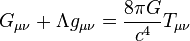# Gravitational wave facts for kids

Kids Encyclopedia Facts

General relativity$G_{\mu \nu} + \Lambda g_{\mu \nu}= {8\pi G\over c^4} T_{\mu \nu}$
Einstein field equations
Phenomena
Kepler problem · Lenses · Waves
Frame-dragging · Geodetic effect
Event horizon · Singularity
Black hole

Gravitational waves are ripples in spacetime which are created whenever objects with mass move. They were predicted by Albert Einstein in 1916 on the basis of his theory of general relativity. As gravitational waves are not created from stationary objects, they must be detected from moving systems. Sources of detectable gravitational waves include binary star systems composed of white dwarfs, neutron stars, or black holes.

On February 11, 2016, the Advanced LIGO team announced that they had directly detected gravitational waves from a pair of black holes merging.

## Gravity and relativity

In physics, gravitational waves are ripples in the curvature of spacetime that travel outward from the source. Albert Einstein predicted them in 1916 on the basis of his theory of general relativity. In theory, gravitational waves transport energy as gravitational radiation.

In general relativity, gravitational waves cannot travel faster than the speed of light. They do not exist in the Newtonian theory of gravitation, in which physical interactions propagate at infinite speed. However, the detection of gravitational waves proves the last prediction of Einstein’s general theory of relativity.

In 1993, the Nobel Prize in Physics was awarded for measurements of the Hulse-Taylor binary star system that suggests gravitational waves are more than mathematical anomalies.

## Images for kidsGravitational wave Facts for Kids. Kiddle Encyclopedia.